UP PREV NEXT

### 4. Mapping to library cells using the patent algorithm

Initial circuit Timing overview Best stage effort Library mapping Gate retiming Input buffering Better accuracy Prior art Summary Conclusions

#### Library analysis to derive the typical load value CS

A library analysis is performed which derives a typical load value CS for each function. This is the load value which can be driven by a gate of size "1". The delay d is then expressed as a function of this typical load and the cell area A. The patent refers to CS as C/S and to A as S.

d = τ × (p + g × COUT /CIN )
d = τ × (p + COUT /(CS×A))

The algorithm works by assigning fixed delays to each gate, so the above expression is reworked so that the cell area can be calculated once the delay d and load capacitance COUT are known.

A = COUT /(CS × ( d/τ - p))

 A is the estimate of the cell area COUT is the load capacitance CS is the typical load value for the function d is the delay fixed during the timing algorithm τ is the characteristic delay of the technology (9.7ps for the 0.13um vsclib) p is the parasitic delay of the gate

CS = CIN /(gA) is calculated for each cell and a single value is then the average over all the cells for each function. The constant timing model sets each cell's delay, and from this an estimate of its area according to the equation above. The area is mapped to a drive strength as shown in the example below for pin a of a 2-XOR gate.

Deriving the drive strength from the estimated cell area, pin a of a 2-XOR gate
CS = 0.47, g = 1.65
x05     x1     x2     x3     x4
CIN    3.34     5.34     10.30     15.07     20.29
Adjusted CIN    2.97     5.34     10.22     16.23     21.23
Actual width    8     8     15     19     25
Est. width A=CIN/(gCS)    3.8     6.9     13.1     20.9     27.4
Mapping  0   x05  5.1 x1 9.5 x2 16.6 x3 23.9 x4

So for example, if the estimated area is 8 tracks, which lies between 5.1 and 9.5, then the mapped cell is the xor2v0x1.

The boundary areas such as 9.5 are determined by the expression
A/6.9 = 13.1/A; A2 = 90.3; A = 9.5

The precise method of getting the drive strength of a cell from its estimated area is not described in the patent. The patent limits itself to describing the use of CS to estimate the area as though this is sufficient for mapping to the standard cell library. The method above uses the logical effort of the individual cell compared to the overall average to adjust the value of CIN. Then an estimated width is calculated from this adjusted CIN using the value of CS. CIN is the slope of line linking the adjusted CIN to the actual area.

A correct mapping is important for the quality of the final netlist timing, and the method used here is an improvement over the one used in the original paper on July 25, 2005.

#### Stage effort ρ=3.6 mapped to vsclib using CS to estimate cell area and hence drive strength

The constant delay model produces a 4-bit adder with the timing shown in the circuits on the right. The top circuit Fig 4a uses a stage effort of 3.6 as does the patent. This gives a critical path of 371ps from pin a(1) to s(3). The maximum input pin capacitance though is 86fF, above the spec of 35fF. The delays and capacitances come from the constant delay model. The drive strengths shown are the nearest available from the library.

The second schematic on the right Fig 4b shows the same netlist as Fig 4a but using the vsclib timing instead of an idealised approximation. There is a good correlation between the estimated delays coming from the constant delay model and the actual library delays. The critical path is slightly down at 368ps from 371ps, and the maximum input capacitance is up from 86fF to 95fF. Although the 4-bit adder timing spec of 350ps is almost met, the input capacitances are too large.

The estimated area of the 4-bit adder is 83.1 gates and the actual area is 85.0 gates, a 2.2% inaccuracy.

#### Stage effort ρ=5.4 mapped to vsclib using CS

The third schematic on the right Fig 4c shows the constant timing delays when a stage effort of 5.4 is used. According to the logical effort theory, if a circuit has the optimum number of logic stages then it is optimally timed if the stage effort of each cell matches the best stage effort, which for the vsclib in 0.13um is 5.4.

The critical path delay is longer, up from 371ps to 441ps, but the input pin capacitances are lower, down from a maximum of 86fF to 45fF.

When the timing from the actual library cells replaces the constant timing in Fig 4d, the delays again remain similar. The critical path is up from 441ps to 449ps and the maximum input capacitance is down slightly from 52fF to 45fF, as shown in the fourth schematic on the right.

The estimated area of the 4-bit adder is 48.7 gates and the actual area is 52.3 gates, a 7% inaccuracy.

These different schematics show the tradeoff that can be made between delay and area.

Critical Path Input Capacitance Gate Count
Initial schematic 545 45 40
Stage effort ρ=5.4 449 45 52
Stage effort ρ=3.6 368 95 85

#### Accuracy considerations

The coefficient CS is a poor fit for estimating the area. The graph below shows the actual mapping of cell width to drive strength for pin a of a 2-XOR gate and the estimated mapping using a single area coefficient CS.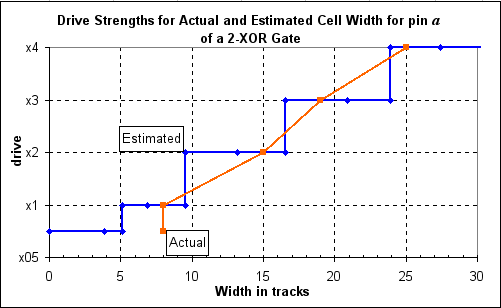This poor fit occurs because a single coefficient CS tries to match the area with the input pin capacitance. A better fit can be made using two coefficients as shown with the curve Est.Area2 in the graph below.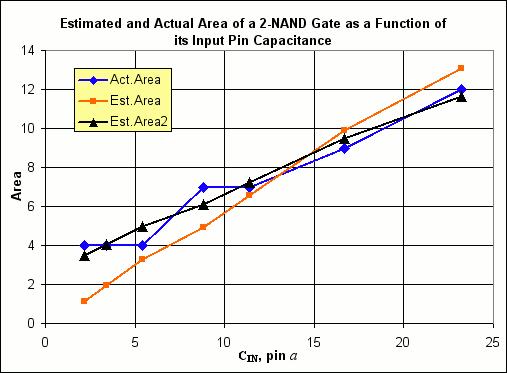Later we will see what difference mapping with two area coefficients makes.

Fig 4a. Adder delays with (i) fixed stage effort of f=3.6; (ii) wireload of 6fF per fanout; (iii) single area coefficient CS to map drive strength; (iv) timing using library averages.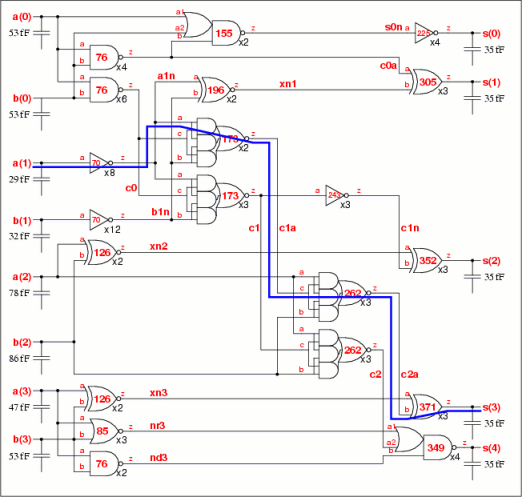Fig 4b. Adder delays with (i) fixed stage effort of f=3.6; (ii) wireload of 6fF per fanout; (iii) single area coefficient CS to map drive strength; (iv) timing from vsclib cells.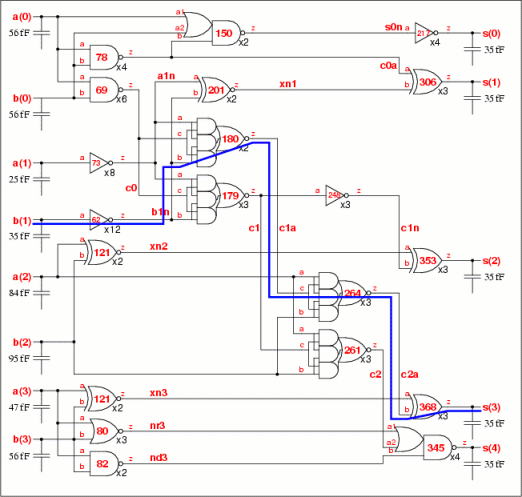Fig 4c. Adder delays with (i) fixed stage effort of f=5.4; (ii) wireload of 6fF per fanout; (iii) single area coefficient CS to map drive strength; (iv) timing using library averages.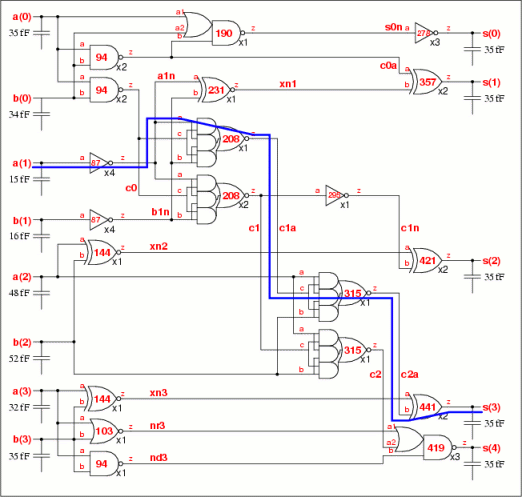Fig 4d. Adder delays with (i) fixed stage effort of f=5.4; (ii) wireload of 6fF per fanout; (iii) single area coefficient CS to map drive strength; (iv) timing from vsclib cells.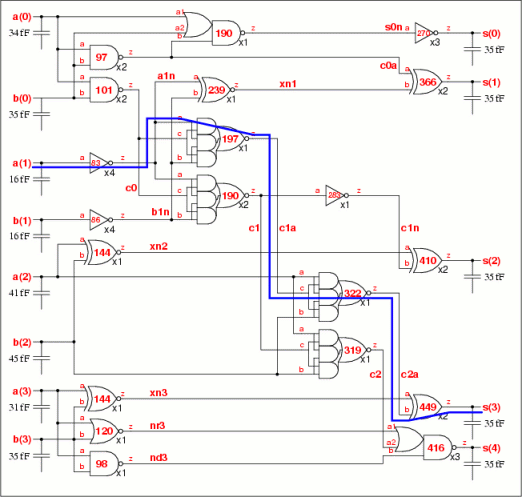UP PREV NEXT 4-AUG-05